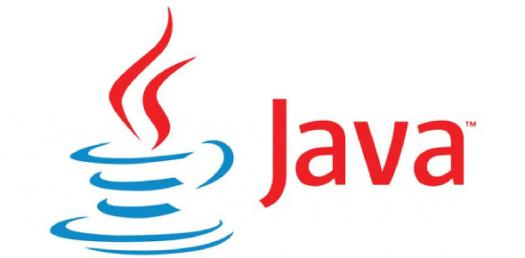# Java: Expression Evaluation Questions! Trivia Quiz

11 Questions | Attempts: 286SettingsThis trivia quiz is made up of expression evaluation questions on Java. It is perfect for any computer geek who wants to test out how good they are when it comes to the solving problems associated with the computer language. How about you try it out and get to perfect your skills in the process? Keep an eye out for more quizzes like it!

• 1.
Write the value of z after the execution of the following code : int j; int z, j=16; z = (4*j++)/3;   Hint: 1)evaluate from Left to Right 2) Prefix(++j) change and use, postfix use and change(j++) 3)Binary operations based on BODMAS rule 4) division, multiplication, and modulus whichever comes first should be performed first.  5) numerator and denominator are integers perform only integer division  151/3 = 50  6) assign the value to z.
• A.

21

• B.

20

• C.

19

• D.

17

• 2.
What will be the value of j and k after execution of the following code:  int j=22,k=20; if(k>=j) k=j;  j=k;
• A.

K=21,j=20

• B.

K=20, j=20

• C.

K=22,j=22

• 3.
Write the value of P after execution of following code : int p; int  k=18; p = (6*++k)/3;
• A.

P=37

• B.

P=38

• C.

P=28

• 4.
What will be displayed in jTextField1 and jTextField2 after the following code is executed: int t; int s; s = 17; t = (4*s++)/2;     jTextField1.setText(" "+t); jTextField2.setText(" "+s);
• A.

30   // jTextField1 17  // jTextField2

• B.

32   // jTextField1 16  // jTextField2

• C.

34   // jTextField1 18  // jTextField2

• D.

Option 4

• 5.
What will be displayed in jTextField1 and jTextField2 after the following code is executed: int num = 35 , num1 = 86 ; jTextField1.setText( num + num1 + " " ) ; jTextField2.setText( " " + num + num1 ) ; Hint :  As you move from left to right, on the left and right of + operator if it is a number, it will add, if anyone is a string it will concatenate
• A.

121   // jTextField1 3586 // jTextField2

• B.

120   // jTextField1 3586 // jTextField2

• C.

121   // jTextField1 3585 // jTextField2

• 6.
Given a String object named 'code' having value as “918” stored in it. What will be the result of the following? JOptionPane.showMessageDialog(null, " " +(code.length( )+ Integer.parseInt(code))); Hint : String code = "918";
• A.

920

• B.

921

• C.

922

• 7.
What will be the content of the jTextArea1 after executing the following code : int n = 99; jTextAreal.setText(Integer.toString(n++)); Hint : Postfix (n++) means use and change
• A.

99

• B.

100

• C.

98

• 8.
Int d; int c; d=15; c = (5*++d)%3+d; Hint : modulus(%) returns the remainder of the division
• A.

18

• B.

17

• C.

16

• 9.
Write the values of sum and x after execution of the following code : int sum, x; sum =1 7; x =1 5; sum = sum + (x++);
• A.

Sum = 30 x=15

• B.

Sum = 32 x=16

• C.

Sum = 31 x=15

• D.

Option 4

• 10.
Write the value that will be assigned to variable C after executing the following statement: C = 125-15*4/3-10+6;
• A.

100

• B.

101

• C.

99

• D.

Option 4

• 11.
Write the values of c and d after execution of following code: int a =1 1; int b = 12; int c; int d; c = ++b; d = a++; c++;
• A.

C=13 d=10

• B.

C=12 d=11

• C.

C=14 d=11

• D.

Option 4

## Related TopicsBack to top
×

Wait!
Here's an interesting quiz for you.